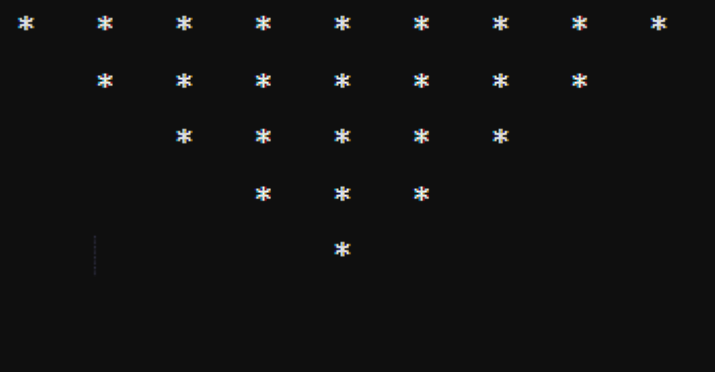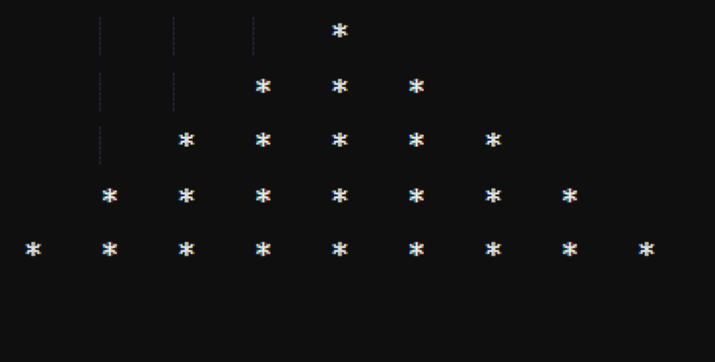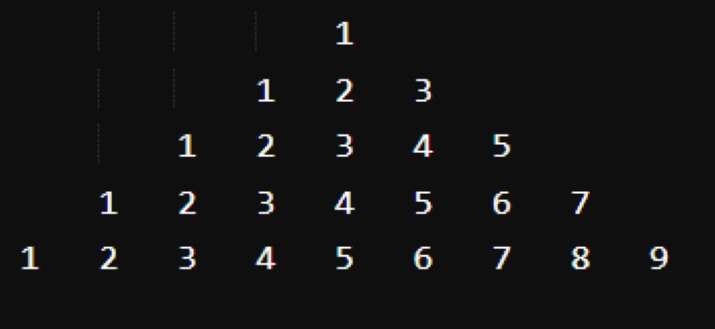# Java Program to Create Pyramid and Pattern

If someone wants to build a strong foundation in Java programming language. Then, it is necessary to understand the working of loops. Also, solving the pyramid pattern problems is the best way to enhance the fundamentals of Java as it includes extensive use of the for and while loops. This article aims to provide a few Java programs to print pyramid patterns with the help of different types of loops available in Java.

## Java Program to Create Pyramid Patterns

We are going to print the following Pyramid Patterns through Java programs −

• Inverted Star Pyramid

• Star Pyramid

• Numeric Pyramid

Let's discuss them one by one.

## Pattern 1: Inverted Star Pyramid### Approach

• Declare and initialize an integer 'n' that specifies number of rows.

• Next, define the initial count of space as 0 and initial star count as 'n + n – 1' so that we can maintain the number of columns as odd.

• Create a nested for loop, the outer one will run till 'n' and the first inner for loop will print the spaces. After printing, we will increment the space count by 1 with each iteration.

• Again take another inner for loop that will print the stars. After printing, we will decrement the star count by 2.

### Example

public class Pyramid1 {
public static void main(String[] args) {
int n = 5;
int spc = 0; // initial space count
int str = n + n - 1; // initial star count
// loop to print the star pyramid
for(int i = 1; i <= n; i++) {
for(int j = 1; j <= spc; j++) { // spaces
System.out.print("\t");
}
spc++; // incrementing spaces
for(int k = 1; k <= str; k++) { // stars
System.out.print("*\t");
}
str -= 2; // decrementing stars
System.out.println();
}
}
}


### Output

*  *  *  *  *  *  *  *  *
*  *  *  *  *  *  *
*  *  *  *  *
*  *  *
*


## Paterrn 2: Star Pyramid### Approach

• Declare and initialize an integer 'n' that specifies number of rows.

• Create a nested for loop, the outer one will run till 'n' and inner for loop will run till number of spaces and print the spaces. After printing, we will decrement the space count by 1.

• Again take another inner for loop that will run till number of stars and print the stars. After printing, we will increment the star count by 2.

### Example

public class Pyramid2 {
public static void main(String[] args) {
int n = 5; // number of rows
int spc = n-1; // initial space count
int str = 1; // initial star count
// loop to print the pyramid
for(int i = 1; i <= n; i++) {
for(int j = 1; j <= spc; j++) { // spaces
System.out.print("\t");
}
spc--; // decrementing spaces
for(int k = 1; k <= str; k++) { // stars
System.out.print("*\t");
}
str += 2; // incrementing stars
System.out.println();
}
}
}


### Output

            *
*  *  *
*	 *  *  *  *
*  *  *  *  *  *  *
*  *  *  *  *  *  *  *  *


## Pattern 3: Numeric Pyramid### Approach

We will use the previous code here, but instead of printing the stars, we will print the column number in each row.

### Example

public class Pyramid3 {
public static void main(String[] args) {
int n = 5; // number of rows
int spc = n-1; // initial space count
int col = 1; // initial column count
// loop to print the pyramid
for(int i = 1; i <= n; i++) {
for(int j = 1; j <= spc; j++) { // spaces
System.out.print("\t");
}
spc--; // decrementing spaces
for(int k = 1; k <= col; k++) { // numbers
System.out.print(k + "\t");
}
col += 2; // incrementing the column
System.out.println();
}
}
}


### Output

            1
1  2  3
1	 2  3  4  5
1  2  3  4  5  6  7
1  2  3  4  5  6  7  8  9


## Conclusion

In this article, we have discussed three Java programs to print pyramid patterns. These pattern solutions will help us to decode the logic of the pattern problems and make us capable of solving other patterns on our own. To solve patterns like these, we use the loops and conditional blocks.

Updated on: 10-Aug-2023

307 Views